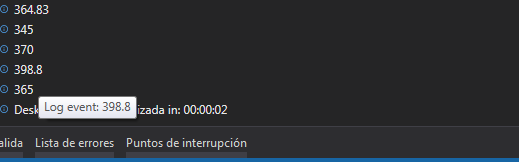# Get minimum value of a variable

415/5000

good morning

-e used a “for each row” to detect the elements
-Then a “switch” to detect a certain element, in this case “costototal”
-which I put in a variable, through the “assign” activity
-this variable has 6 “6 numbers” data
-I would like to detect the smallest of them
-Put the term (if any?), in an activity “write line” and have the lowest number

thank you

that’s the for each and the variable “costototal”those are the data of the variable “costototal”, I would like to have a way of knowing the minimum value

If you only want the smallest value of a datatable column, you can just use Sort Activity and then get the value of the first row, dont need to For Each Row…

1 Like

Hi @borismh
Use Bala Rev Activities or use the dT Query

intminvalue=Convert.ToInt32(dt.AsEnumerable().Max(Function(row) row(“Column2”)))

Thanks
Ashwin S

Please read the question, he wants minimum and not maximum, also his value is not int32 he would be loosing precision there…

Hi @bcorrea

Sorry i didnt c that

intminvalue=Convert.ToInt32(dt.AsEnumerable().Min(Function(row) row(“Column2”)))Thanks
Ashwin S

still Convert.ToInt32 is not the answer…is that first I need certain excel values, since if I use that method that they tell me I would get the minimum value of the entire column, that’s why I use “for each” and then the “switch”

lol, that is a weird excel fileso create a variable of type List(Of Double) and in every loop you add the value in the collection, after you can get it like this:

``````Dim RowindexMin As Double = (From row As RowsFound In MyList.OrderBy(Function(x) x.LineValue) Select row.RowIndex).First
``````
1 Like

@bcorrea
Sorry I didn’t understand, could you explain me

@bcorrea
excuse me could you explain to me, I get “expected end of expression”

Before the loop you assign: MyList = new List(Of Double)
Inside the loop you add the values using a Add To Collection activity.
After the end of loop you assign:
MySmallValue = MyList.ToArray.Min()

3 Likes

@bcorrea
thank you, it came out

1 Like

This topic was automatically closed 3 days after the last reply. New replies are no longer allowed.